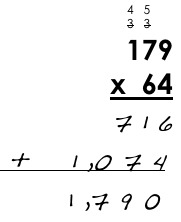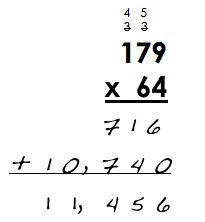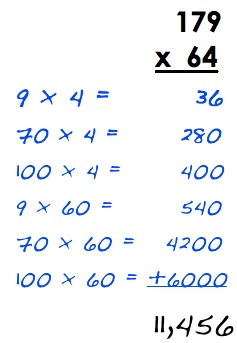# Elmer's Multiplication Error

Alignments to Content Standards: 5.NBT.B.5

This is Elmer’s work on a multiplication problem:1. Use estimation to explain why Elmer’s answer is not reasonable.
2. What error do you think Elmer made? Why do you think he made that error?
3. Find $179 \times 64$ using a correct version of Elmer's method. Then show another way of doing it to help Elmer see why your answer is correct.

## IM Commentary

This task has students explore a very common multiplication error that occurs when using the standard algorithm. Often, students are not thinking about the meaning of a particular digit based on its place in the number, and in fact, that is one of the advantages of the algorithm--you only need single-digit multiplication facts to use it. However, it is easy to forget a step or misalign the addends if you aren't thinking about the value of a particular digit. This task is designed to help students catch these kinds of errors. Part (a) asks them to think about the reasonableness of an answer and part (b) asks them to analyze the error. Part (c) helps students see and explain what went wrong and also helps them develop flexibility in solving multi-digit multiplication problems, which is an aspect of fluency.

## Solution

a. 179 is greater than 100 and 64 is greater than 60, and $100 \times 60 = 6,000$. Since $179 \times 64$ is greater than $100 \times 60$, we can see that Elmer's answer of 1,790 is much too small.

b. The standard algorithm breaks  $64 \times 179$ into  $60 \times 179 + 4 \times 179$. Elmer's work for $4 \times 179$ is correct $(4 \times 179 = 716).$ However, on the next line, he wrote  $6 \times 179$ instead of  $60 \times 179$. This is why his answer is much too small.

c. Here is the correct calculation using Elmer's method:Here is the answer using the partial products algorithm:Here is a rectangle with side lengths $100+70+9$ and $60+4$ that shows all of the partial products as the area of part of the rectangle: